International
Tables for
Crystallography
Volume B
Reciprocal space
Edited by U. Shmueli

International Tables for Crystallography (2010). Vol. B, ch. 2.5, pp. 375-376

## Section 2.5.7.1. Formation of projection images in single-particle reconstruction

P. A. Penczekg

#### 2.5.7.1. Formation of projection images in single-particle reconstruction

| top | pdf |

Cryo-electron microscopy (cryo-EM) in combination with the single-particle approach is a new method of structure determination for large macromolecular assemblies. Currently, resolution in the range 10 to 30 Å can be reached routinely, although in a number of pilot studies it has been possible to obtain structures at 4 to 8 Å. Theoretically, electron microscopy can yield data exceeding atomic resolution, but the difficulties in overcoming the very low signal-to-noise ratio (SNR) and low contrast in the data, combined with the adverse effects of the contrast transfer function (CTF) of the microscope, hamper progress in fulfilling the potential of the technique. However, in recent years, cryo-EM has proven its power in the structure determination of large macromolecular assemblies and machines which are too large and complex for the more traditional techniques of structural biology, i.e., X-ray crystallography and NMR spectroscopy.

Single-particle reconstruction is based on the assumption that a protein exists in solution in multiple copies of the same basic structure. Unlike in crystallography, no ordering of the structure within a crystal grid is required; the enhancement of the SNR is achieved by bringing projection images of different (but structurally identical) proteins into register and averaging them. This is why the technique is sometimes called `crystallography without crystals'.

Within the linear weak-phase-object approximation of the image formation process in the microscope [see equation (2.5.2.43)in Section 2.5.2], 2D projections represent line integrals of the Coulomb potential of the particle under examination convoluted with the point-spread function of the microscope, s, as introduced in Section 2.5.1. In addition, we have to consider the translation t of the projection in the plane of micrograph, suppression of high-frequency information by the envelope function E of the microscope, and two additive noises mB and mS . The first one is a coloured background noise, while the second is attributed to the residual scattering by the solvent or the supporting thin layer of carbon, if used, assumed to be white and affected by the transfer function of the microscope in the same way as the imaged protein. In order to have the image formation model correspond more closely to the physical reality of data collection, we write equation (2.5.6.4)from Section 2.5.6such that the projection operation is always realized in the z direction of the coordinate system (corresponding to the direction of propagation of the electron beam), while the molecule is rotated arbitrarily by three Eulerian angles: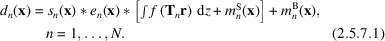Here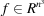represents the three-dimensional (3D) electron density of the imaged macromolecule and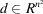is the nth observed two-dimensional (2D) projection image. The total number of projection images N depends on the structure determination project, and can vary from a few hundred to hundreds of thousands. Further, e is the inverse Fourier transform of the envelope function,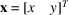is a vector of coordinates in the plane of projections,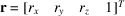is a vector of coordinates associated with nth macromolecule,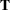is the 4 × 4 transformation matrix given by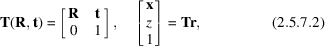with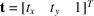being the shift vector of translation of the object (and its projection) in the xy plane (translation in z is irrelevant due to the projection operation) and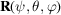is the 3 × 3 rotation matrix specified by three Eulerian angles. As in Section 2.5.6, two of the angles define the direction of projection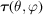, while the third angle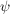results in rotation of the projection image in the plane of the formed image xy; changing this angle does not provide any additional information about the structure f. Both types of noise are assumed to be mutually uncorrelated and independent between projection images (i.e.,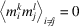; k, l = S, B) and also uncorrelated with the signal (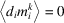; k = S, B). Model (2.5.7.1)is semi-empirical in that, unlike in the standard model, we have two contributions to the noise. Although in principle amorphous ice should not be affected by the CTF, so the term mS should be absorbed into mB, in practice the buffer in which the protein is purified is not pure water and it is possible to observe CTF effects by imaging frozen buffer alone. Moreover, if a thin support carbon is used, it will be a source of very strong CTF-affected noise also included in mB.

In Fourier space, (2.5.7.1)is written by taking advantage of the central section theorem [equation (2.5.6.8)of Section 2.5.6]: the Fourier transform of a projection is extracted as a Fourier plane uv of a rotated Fourier transform of a 3D object: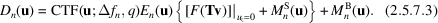The capital letters denote Fourier transforms of objects appearing in (2.5.7.1)while CTF (a Fourier transform of s) depends, among other parameters that are set very accurately (such as the accelerating voltage of the microscope), on the defocus setting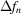and the amplitude contrast ratio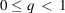that reflects the presence of the amplitude contrast that is due to the removal of widely scattered electrons [the real term in (2.5.5.14)]. For the range of frequency considered, q is assumed to be constant and the CTF is written in terms of the phase perturbation function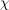[given by equation (2.5.2.33)] as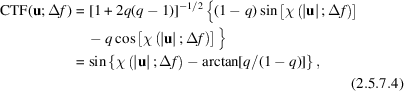where for simplicity we assumed no astigmatism. Finally, the rotationally averaged power spectrum of the observed image, calculated as the expectation value of its squared Fourier intensities (2.5.7.3), is given by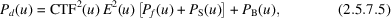where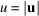is the modulus of spatial frequency.